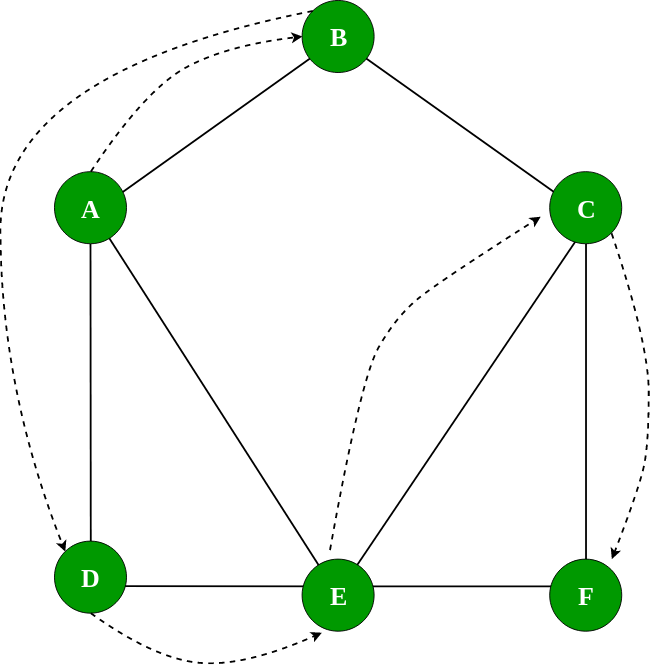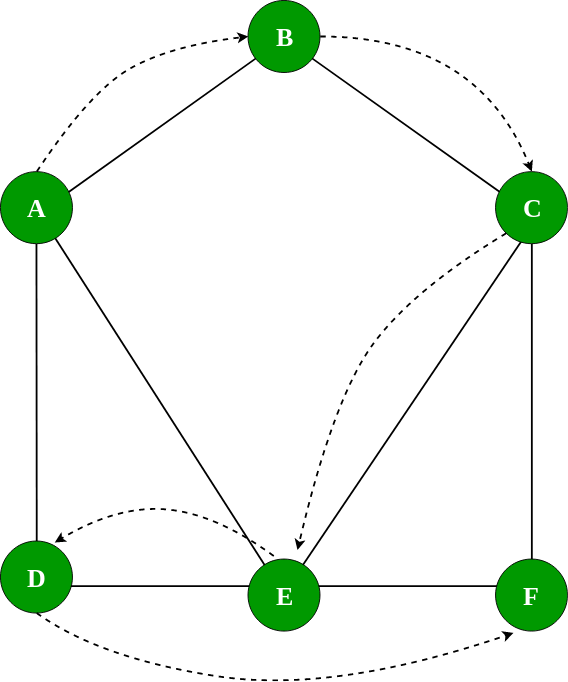# Implementation of Graph in JavaScript

In this article we would be implementing the Graph data structure in JavaScript. Graph is a non-linear data structure. A graph G contains a set of vertices V and set of Edges E. Graph has lots of application in computer science.
Graph is basically divided into two broad categories :

• Directed Graph (Di- graph) – Where edges have direction.
• Undirected Graph – Where edges do not represent any directed

There are various ways to represent a Graph :-

There are several other ways like incidence matrix, etc. but these two are most commonly used. Refer to Graph and its representations for the explaination of Adjacency matrix and list.

In this article, we would be using Adjacency List to represent a graph because in most cases it has a certain advantage over the other representation.
Now Lets see an example of Graph class-

 `// create a graph class ` `class` `Graph { ` `    ``// defining vertex array and ` `    ``// adjacent list ` `    ``constructor(noOfVertices) ` `    ``{ ` `        ``this``.noOfVertices = noOfVertices; ` `        ``this``.AdjList = ``new` `Map(); ` `    ``} ` ` `  `    ``// functions to be implemented ` ` `  `    ``// addVertex(v) ` `    ``// addEdge(v, w) ` `    ``// printGraph() ` ` `  `    ``// bfs(v) ` `    ``// dfs(v) ` `} `

The above example shows a framework of Graph class. We define two private variable i.e noOfVertices to store the number of vertices in the graph and AdjList, which stores a adjacency list of a particular vertex. We used a Map Object provided by ES6 in order to implement Adjacency list. Where key of a map holds a vertex and values holds an array of an adjacent node.

Now let’s implement functions to perform basic operations on the graph:

1. addVertex(v) – It adds the vertex v as key to adjList and initialize its values with an array.
 `// add vertex to the graph ` `addVertex(v) ` `{ ` `    ``// initialize the adjacent list with a ` `    ``// null array ` `    ``this``.AdjList.set(v, []); ` `} `

2. addEdge(src, dest) – It adds an edge between the src and dest.
 `// add edge to the graph ` `addEdge(v, w) ` `{ ` `    ``// get the list for vertex v and put the ` `    ``// vertex w denoting edge betweeen v and w ` `    ``this``.AdjList.get(v).push(w); ` ` `  `    ``// Since graph is undirected, ` `    ``// add an edge from w to v also ` `    ``this``.AdjList.get(w).push(v); ` `} `

In order to add edge we get the adjacency list of the corresponding src vertex and add the dest to the adjacency list.

3. printGraph() – It prints vertices and its adjacency list.
 `// Prints the vertex and adjacency list ` `printGraph() ` `{ ` `    ``// get all the vertices ` `    ``var get_keys = ``this``.AdjList.keys(); ` ` `  `    ``// iterate over the vertices ` `    ``for` `(var i of get_keys)  ` `{ ` `        ``// great the corresponding adjacency list ` `        ``// for the vertex ` `        ``var get_values = ``this``.AdjList.get(i); ` `        ``var conc = ``""``; ` ` `  `        ``// iterate over the adjacency list ` `        ``// concatenate the values into a string ` `        ``for` `(var j of get_values) ` `            ``conc += j + ``" "``; ` ` `  `        ``// print the vertex and its adjacency list ` `        ``console.log(i + ``" -> "` `+ conc); ` `    ``} ` `} `

Lets see an example of a graphNow we will use the graph class to implement the graph shown above:

 `// Using the above implemented graph class ` `var g = ``new` `Graph(``6``); ` `var vertices = [ ``'A'``, ``'B'``, ``'C'``, ``'D'``, ``'E'``, ``'F'` `]; ` ` `  `// adding vertices ` `for` `(var i = ``0``; i < vertices.length; i++) { ` `    ``g.addVertex(vertices[i]); ` `} ` ` `  `// adding edges ` `g.addEdge(``'A'``, ``'B'``); ` `g.addEdge(``'A'``, ``'D'``); ` `g.addEdge(``'A'``, ``'E'``); ` `g.addEdge(``'B'``, ``'C'``); ` `g.addEdge(``'D'``, ``'E'``); ` `g.addEdge(``'E'``, ``'F'``); ` `g.addEdge(``'E'``, ``'C'``); ` `g.addEdge(``'C'``, ``'F'``); ` ` `  `// prints all vertex and ` `// its adjacency list ` `// A -> B D E ` `// B -> A C ` `// C -> B E F ` `// D -> A E ` `// E -> A D F C ` `// F -> E C ` `g.printGraph(); `

Graph Traversal

We will implement the most common graph traversal algorithm:

Implementation of BFS and DFS:

1. bfs(startingNode) – It performs Breadth First Search from the given startingNode
 `// function to performs BFS ` `bfs(startingNode) ` `{ ` ` `  `    ``// create a visited array ` `    ``var visited = []; ` `    ``for` `(var i = ``0``; i < ``this``.noOfVertices; i++) ` `        ``visited[i] = ``false``; ` ` `  `    ``// Create an object for queue ` `    ``var q = ``new` `Queue(); ` ` `  `    ``// add the starting node to the queue ` `    ``visited[startingNode] = ``true``; ` `    ``q.enqueue(startingNode); ` ` `  `    ``// loop until queue is element ` `    ``while` `(!q.isEmpty()) { ` `        ``// get the element from the queue ` `        ``var getQueueElement = q.dequeue(); ` ` `  `        ``// passing the current vertex to callback funtion ` `        ``console.log(getQueueElement); ` ` `  `        ``// get the adjacent list for current vertex ` `        ``var get_List = ``this``.AdjList.get(getQueueElement); ` ` `  `        ``// loop through the list and add the element to the ` `        ``// queue if it is not processed yet ` `        ``for` `(var i in get_List) { ` `            ``var neigh = get_List[i]; ` ` `  `            ``if` `(!visited[neigh]) { ` `                ``visited[neigh] = ``true``; ` `                ``q.enqueue(neigh); ` `            ``} ` `        ``} ` `    ``} ` `} `

In the above method we have implemented the BFS algorithm. A Queue is used to keep the unvisited nodes
Lets use the above method and traverse along the graph

 `// prints ` `// BFS ` `// A B D E C F ` `console.log(``"BFS"``); ` `g.bfs(``'A'``); `

The Diagram below shows the BFS on the example graph:2. dfs(startingNode) – It performs the Depth first traversal on a graph
 `// Main DFS method ` `dfs(startingNode) ` `{ ` ` `  `    ``var visited = []; ` `    ``for` `(var i = ``0``; i < ``this``.noOfVertices; i++) ` `        ``visited[i] = ``false``; ` ` `  `    ``this``.DFSUtil(startingNode, visited); ` `} ` ` `  `// Recursive function which process and explore ` `// all the adjacent vertex of the vertex with which it is called ` `DFSUtil(vert, visited) ` `{ ` `    ``visited[vert] = ``true``; ` `    ``console.log(vert); ` ` `  `    ``var get_neighbours = ``this``.AdjList.get(vert); ` ` `  `    ``for` `(var i in get_neighbours) { ` `        ``var get_elem = get_neighbours[i]; ` `        ``if` `(!visited[get_elem]) ` `            ``this``.DFSUtil(get_elem, visited); ` `    ``} ` `} `

In the above example dfs(startingNode) is used to initialize a visited array and DFSutil(vert, visited)
contains the implementation of DFS algorithm
Lets use the above method to traverse along the graph

 `// prints ` `// DFS ` `// A B C E D F ` `console.log(``"DFS"``); ` `g.dfs(``'A'``); `

The Diagram below shows the DFS on the example graph## tags:

Graph JavaScript JavaScript-DS Graph